# Complex structure

(diff) ← Older revision | Latest revision (diff) | Newer revision → (diff)
A complex structure on a real vector spaceis the structure of a complex vector space onthat is compatible with the original real structure. The complex structure onis completely determined by the operator of multiplication by the number, the role of which can be taken by an arbitrary linear transformationsatisfying, whereis the identity. Therefore, a transformation of this type is often called a complex structure on. Ifis endowed with a complex structure andis a basis of this space over, thenforms a basis of it over, so that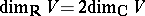. Ifis a complex structure onthen the complexification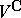ofdecomposes into a direct sum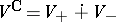, whereare the eigen spaces of the transformationextended tocorresponding to the eigen values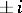, and. Conversely, each complex subspace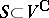such that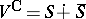determines a complex structure onfor which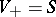.
Any two complex structures on a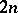-dimensional real spacecan be mapped into each other by some automorphism of. The set of all complex structures onis thus a homogeneous space of the groupand is identified with the quotient space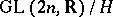, where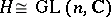is the subgroup of non-singular matrices of the form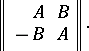A complex structure on a differentiable manifold is the structure of a complex-analytic manifold (cf. Analytic manifold). Ifis a differentiable manifold, then a complex structure onis a complex-analytic atlas onthat is compatible with the real differentiable atlas defined on. Here. A complex structure oninduces a complex structure on each tangent space, and therefore induces onan almost-complex structure which completely determines it.